# Constructing Line Graphs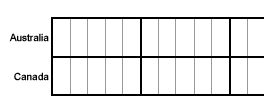In the last lesson, we learned that a line graph is useful for displaying data or information that changes continuously over time. We read and interpreted information from various line graphs. However, we did not make any line graphs of our own. In this lesson, we will show you the steps for constructing a line graph.

Example 1: The table below shows Jill's math scores in secondary school (grades 7 through 12). Construct a line graph to visually display this data.

 Jill's Secondary Math Scores Grade Level Math Score (%) 7 72 8 75 9 81 10 80 11 83 12 91

 Step 1: Find the range in values. There are two sets of values. What units are used? What is the greatest value and the least value for the first set? What is the greatest value and the least value for the second set?

The units are grade level and math score (%). The grade level ranges from 7 to 12 and the math score ranges from 72 to 91.

 Step 2: Determine a Scale. Start with the horizontal scale. If you are using graph paper, let 1 unit on the graph paper equal 1 unit of the values you are graphing. Determine whether the greatest value will fit on the graph. If it doesn't, then change the scale and try again. Now repeat this process for the vertical scale.

The vertical scale must start at 0. So the vertical scale will range from 0 to 100.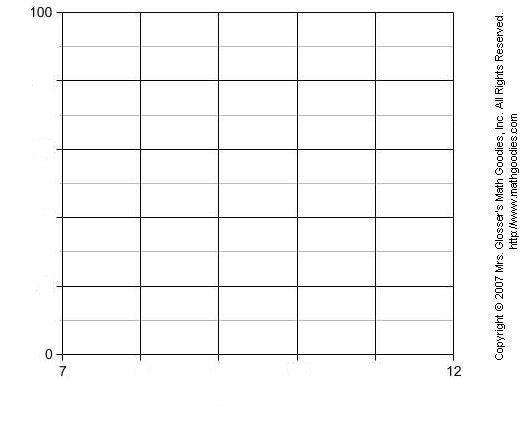Step 3: Label the graph. Mark each unit across the horizontal scale and along the vertical scale. Label the marks by the units they represent.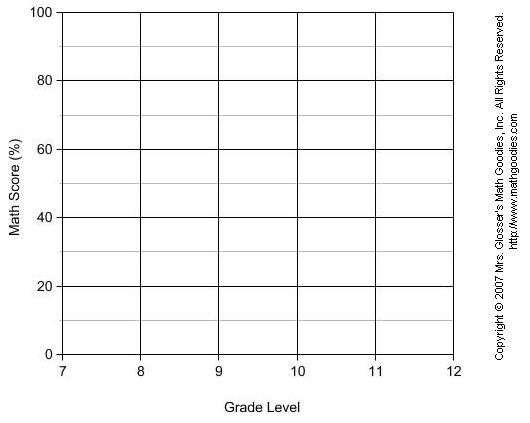Step 4: Plot the points and connect them. Plot a point for each pair of values. Which item of a pair is indicated by the horizontal scale? by the vertical scale? How many points will you plot? Connect the points with straight lines from left to right.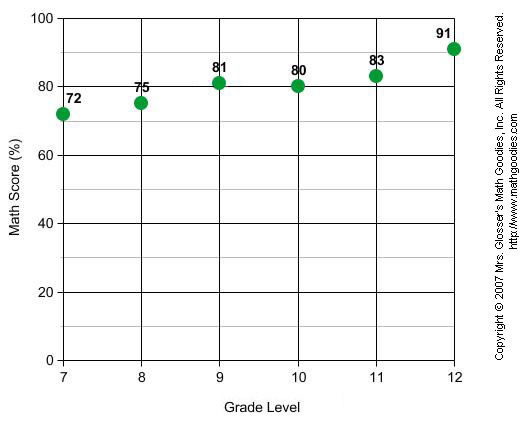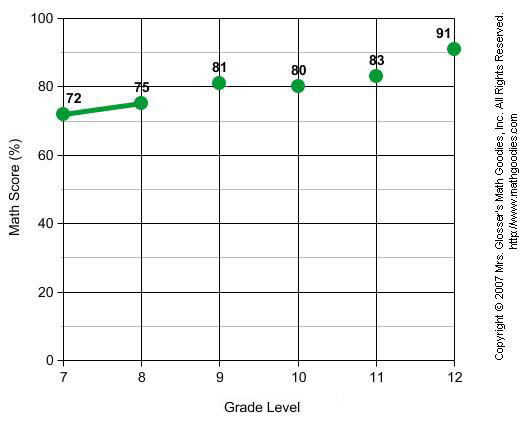Step 5: Give the graph a title. What is your graph about?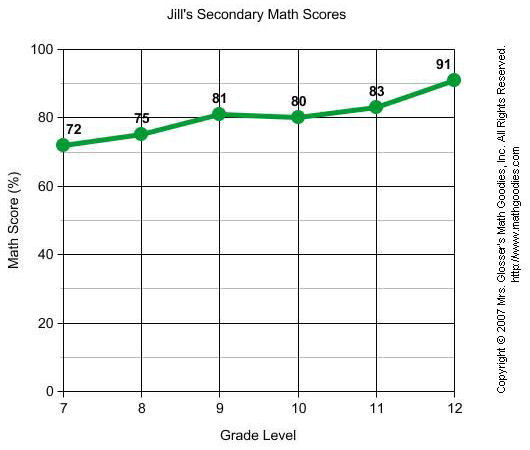In Example 1, we wrote that the vertical scale must start at 0. Let's take a look at why this is so. Compare the line graph from Example 1 with the line graph below. The vertical scale in the graph below starts at 70. This makes the math scores appear to change much faster than they did in Example 1. Thus, by starting the vertical scale at 70, this graph is misleading.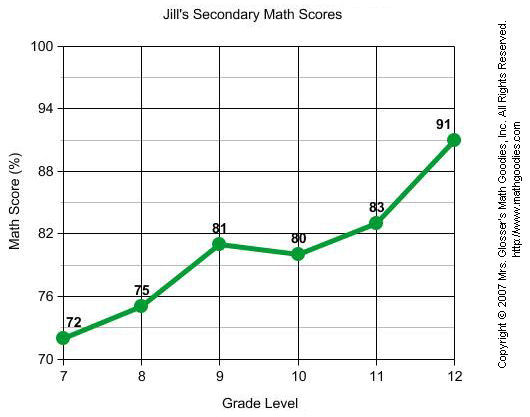Example 2: Anybank published its interest rates each day for 7 days as shown in the table below. Construct a line graph to visually display this data.

 Anybank Interest Rates Day Interest Rate (%) 1 1.500 2 1.475 3 1.375 4 1.275 5 1.125 6 1.300 7 1.100

 Step 1: Find the range in values. There are two sets of values. What units are used? What is the greatest value and the least value for the first set? What is the greatest value and the least value for the second set?

The units are day and interest rate (%). The day ranges from 1 to 7 and the interest rate ranges from 1.1 to 1.5.

 Step 2: Determine a Scale. Start with the horizontal scale. If you are using graph paper, let 1 unit on the graph paper equal 1 unit of the values you are graphing. Determine whether the greatest value will fit on the graph. If it doesn't, then change the scale and try again. Now repeat this process for the vertical scale.

The vertical scale must start at 0. So, the vertical scale will range from 0 to 1.5.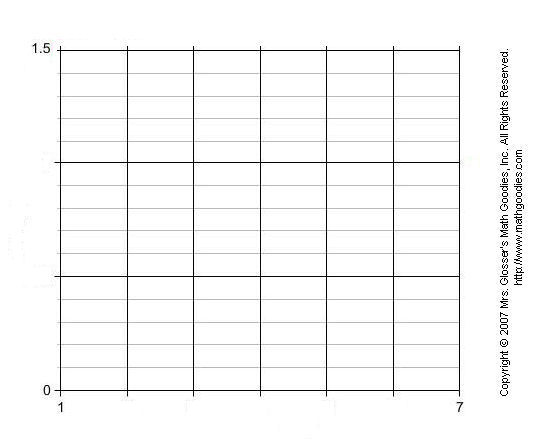Step 3: Label the graph. Mark each unit across the horizontal scale and along the vertical scale. Label the marks by the units they represent.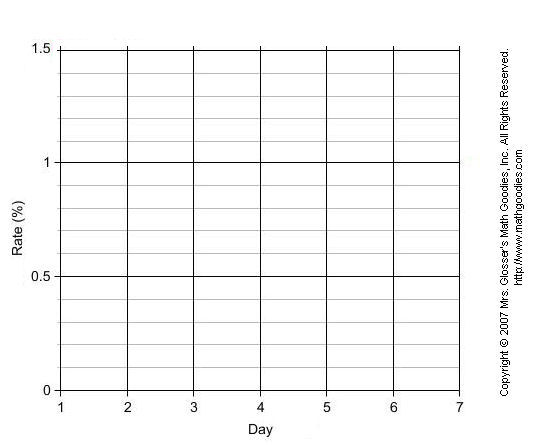Step 4: Plot the points and connect them. Plot a point for each pair of values. Which item of a pair is indicated by the horizontal scale? by the vertical scale? How many points will you plot? Connect the points with straight lines from left to right.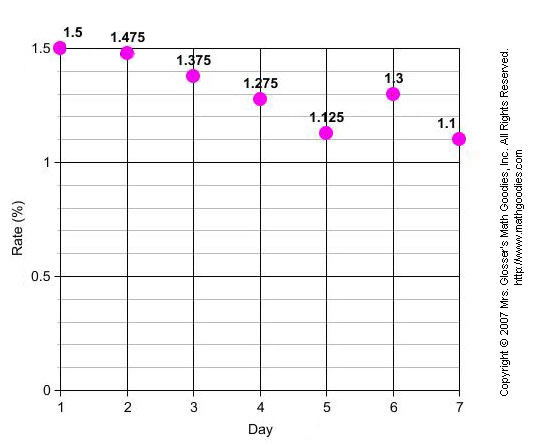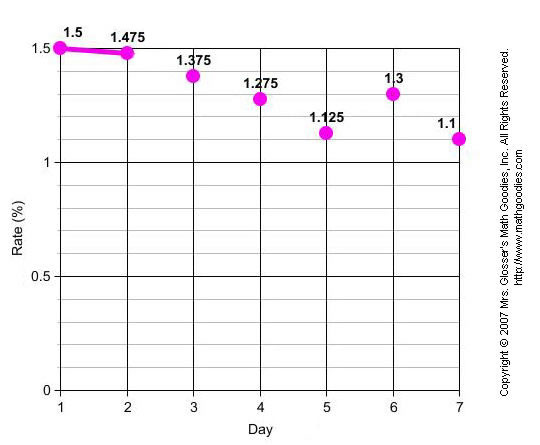Step 5: Give the graph a title. What is your graph about?Summary: There are 5 steps for constructing a line graph. These steps are listed below.

 Procedure for Constructing a Line Graph Step 1: Find the range in values. Step 2: Determine a scale. Step 3: Label the graph. Step 4: Plot the points and connect them. Step 5: Give the graph a title.

### Exercises

Directions: Use the procedure above to construct a line graph for each table given in the exercises below. You may print and use our line graph paper (PDF).

Exercise 1: In 2000, a law was passed against the use of cell phones while driving in Anytown, N.Y. The number of people who use cell phones while driving in Anytown has changed each year since then as shown in the table below. Construct a line graph to visually display this data.

 Cell Phone Use While Driving in Anytown, NY Year Number of People 2000 309 2001 274 2002 256 2003 238 2004 197 2005 203 2006 195 2007 192

Solution: Answers will vary depending on the scales used. Click here to see a sample line graph for Exercise 1.

Exercise 2: There were a total of 7 competitions for the 50-Meter Dash at a school. Winning times were recorded in seconds as shown in the table below. Construct a line graph to visually display this data.

 50-Meter Dash Competition Winning Time (seconds) First 8.7 Second 11.6 Third 10.9 Fourth 12.7 Fifth 13.1 Sixth 9.5 Seventh 14.4

Solution: Answers will vary depending on the scales used. Click here to see a sample line graph for Exercise 2.